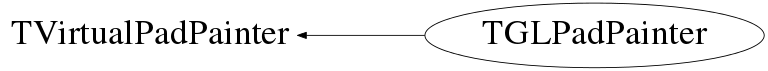```"Delegating" part of TGLPadPainter. Line/fill/etc. attributes can be
set inside TPad, but not only there:
many of them are set by base sub-objects of 2d primitives
(2d primitives usually inherit TAttLine or TAttFill etc.).  And these sub-objects
call gVirtualX->SetLineWidth ... etc. So, if I save some attributes in my painter,
it will be mess - at any moment I do not know, where to take line attribute - from
gVirtualX or from my own member. So! All attributed, _ALL_ go to/from gVirtualX.
```

public:
private:

## Data Members

public:
 enum TVirtualPadPainter::EBoxMode { kHollow kFilled }; enum TVirtualPadPainter::ETextMode { kClear kOpaque };
private:
 TGLFont fF TGLFontManager fFM Bool_t fIsHollowArea Rgl::Pad::GLLimits fLimits Bool_t fLocked Rgl::Pad::MarkerPainter fMarker vector fPoly Rgl::Pad::PolygonStippleSet fSSet Rgl::Pad::Tesselator fTess Int_t fVp vector fVs Vertex buffer for tesselator.

## Class Charts## Function documentation

Color_t GetLineColor() const
```Delegate to gVirtualX.
```
Style_t GetLineStyle() const
```Delegate to gVirtualX.
```
Width_t GetLineWidth() const
```Delegate to gVirtualX.
```
void SetLineColor(Color_t lcolor)
```Delegate to gVirtualX.
```
void SetLineStyle(Style_t lstyle)
```Delegate to gVirtualX.
```
void SetLineWidth(Width_t lwidth)
```Delegate to gVirtualX.
```
Color_t GetFillColor() const
```Delegate to gVirtualX.
```
Style_t GetFillStyle() const
```Delegate to gVirtualX.
```
Bool_t IsTransparent() const
```Delegate to gVirtualX.
IsTransparent is implemented as inline function in TAttFill.
```
void SetFillColor(Color_t fcolor)
```Delegate to gVirtualX.
```
void SetFillStyle(Style_t fstyle)
```Delegate to gVirtualX.
```
void SetOpacity(Int_t percent)
```Delegate to gVirtualX.
```
Short_t GetTextAlign() const
```Delegate to gVirtualX.
```
Float_t GetTextAngle() const
```Delegate to gVirtualX.
```
Color_t GetTextColor() const
```Delegate to gVirtualX.
```
Font_t GetTextFont() const
```Delegate to gVirtualX.
```
Float_t GetTextSize() const
```Delegate to gVirtualX.
```
Float_t GetTextMagnitude() const
```Delegate to gVirtualX.
```
void SetTextAlign(Short_t align)
```Delegate to gVirtualX.
```
void SetTextAngle(Float_t tangle)
```Delegate to gVirtualX.
```
void SetTextColor(Color_t tcolor)
```Delegate to gVirtualX.
```
void SetTextFont(Font_t tfont)
```Delegate to gVirtualX.
```
void SetTextSize(Float_t tsize)
```Delegate to gVirtualX.
```
void SetTextSizePixels(Int_t npixels)
```Delegate to gVirtualX.
```

```Not required at the moment.
```
void ClearDrawable()
```Not required at the moment.
```
void CopyDrawable(Int_t id, Int_t px, Int_t py)
```Not required at the moment.
```
void DestroyDrawable()
```Not required at the moment.
```
void SelectDrawable(Int_t device)
```For gVirtualX this means select pixmap (or window)
and all subsequent drawings will go into
this pixmap. For OpenGL this means the change of
coordinate system and viewport.
```
void InitPainter()
```Init gl-pad painter:
1. 2D painter does not use depth test, should not modify
depth-buffer content (except initial cleanup).
2. Disable cull face.
3. Disable lighting.
4. Set viewport (to the whole canvas area).
5. Set camera.
6. Unlock painter.
```
void InvalidateCS()
```When TPad::Range for gPad is called, projection
must be changed in OpenGL.
```
void LockPainter()
```Locked state of painter means, that
GL context can be invalid, so no GL calls
can be executed.
```
void DrawLine(Double_t x1, Double_t y1, Double_t x2, Double_t y2)
```Draw line segment.
```
void DrawLineNDC(Double_t u1, Double_t v1, Double_t u2, Double_t v2)
```Draw line segment in NDC coordinates.
```
void DrawBox(Double_t x1, Double_t y1, Double_t x2, Double_t y2, TVirtualPadPainter::EBoxMode mode)
```Draw filled or hollow box.
```
void DrawFillArea(Int_t n, const Double_t* x, const Double_t* y)
```Draw tesselated polygon (probably, outline only).
```
void DrawFillArea(Int_t n, const Float_t* x, const Float_t* y)
```Draw tesselated polygon (never called, probably, since TPad::PaintFillArea for floats
is deprecated).
```
void DrawPolyLine(Int_t n, const Double_t* x, const Double_t* y)
```Draw poly-line in user coordinates.
```
void DrawPolyLine(Int_t n, const Float_t* x, const Float_t* y)
```Never called?
```
void DrawPolyLineNDC(Int_t n, const Double_t* u, const Double_t* v)
```Poly line in NDC.
```
void DrawPolyMarker(Int_t n, const Double_t* x, const Double_t* y)
```Poly-marker.
```
void DrawPolyMarker(Int_t n, const Float_t* x, const Float_t* y)
```Poly-marker.
```
void DrawPolyMarker()
```Poly-marker.
```
void DrawText(Double_t x, Double_t y, const char* text, TVirtualPadPainter::ETextMode mode)
```Draw text. This operation is especially
dangerous if in locked state -
ftgl will assert on zero texture size
(which is result of bad GL context).
```
void DrawText(Double_t , Double_t , const wchar_t* , TVirtualPadPainter::ETextMode )
```Draw text. This operation is especially
dangerous if in locked state -
ftgl will assert on zero texture size
(which is result of bad GL context).
```
void DrawTextNDC(Double_t x, Double_t y, const char* text, TVirtualPadPainter::ETextMode mode)
```Draw text in NDC. This operation is especially
dangerous if in locked state -
ftgl will assert on zero texture size
(which is result of bad GL context).
```
void DrawTextNDC(Double_t , Double_t , const wchar_t* , TVirtualPadPainter::ETextMode )
```Draw text in NDC. This operation is especially
dangerous if in locked state -
ftgl will assert on zero texture size
(which is result of bad GL context).
```
void SaveProjectionMatrix() const
```Save the projection matrix.
Attention! GL_PROJECTION will become the current matrix
after this call!
```
void RestoreProjectionMatrix() const
```Restore the projection matrix.
Attention! GL_PROJECTION will become the current matrix
after this call!
```
void SaveModelviewMatrix() const
```Save the modelview matrix.
Attention! GL_MODELVIEW will become the current matrix
after this call!
```
void RestoreModelviewMatrix() const
```Restore the modelview matrix.
Attention! GL_MODELVIEW will become the current matrix
after this call!
```
void SaveViewport()
```Extract and save the current viewport.
```
void RestoreViewport()
```Restore the saved viewport.
```
``` Using TImage save frame-buffer contents as a picture.
```At the moment I assume both linear and radial gradients will work the same way  -